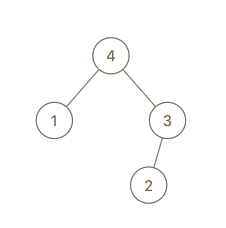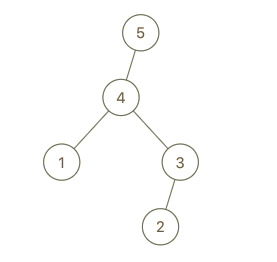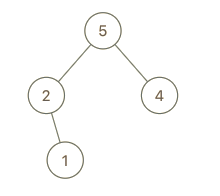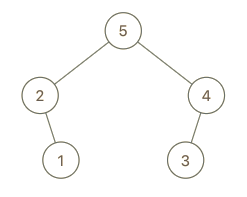##### Welcome to Subscribe On Youtube

Formatted question description: https://leetcode.ca/all/998.html

# 998. Maximum Binary Tree II (Medium)

We are given the root node of a maximum tree: a tree where every node has a value greater than any other value in its subtree.

Just as in the previous problem, the given tree was constructed from an list A (root = Construct(A)) recursively with the following Construct(A) routine:

• If A is empty, return null.
• Otherwise, let A[i] be the largest element of A.  Create a root node with value A[i].
• The left child of root will be Construct([A, A, ..., A[i-1]])
• The right child of root will be Construct([A[i+1], A[i+2], ..., A[A.length - 1]])
• Return root.

Note that we were not given A directly, only a root node root = Construct(A).

Suppose B is a copy of A with the value val appended to it.  It is guaranteed that B has unique values.

Return Construct(B).

Example 1:Input: root = [4,1,3,null,null,2], val = 5
Output: [5,4,null,1,3,null,null,2]
Explanation: A = [1,4,2,3], B = [1,4,2,3,5]


Example 2:Input: root = [5,2,4,null,1], val = 3
Output: [5,2,4,null,1,null,3]
Explanation: A = [2,1,5,4], B = [2,1,5,4,3]


Example 3:Input: root = [5,2,3,null,1], val = 4
Output: [5,2,4,null,1,3]
Explanation: A = [2,1,5,3], B = [2,1,5,3,4]


Note:

1. 1 <= B.length <= 100

Companies:

Related Topics:
Tree

Similar Questions:

## Solution 1.

• Find insertion point: Keep repeating the following logic until node becomes NULL
• If val < node->val, go right.
• Otherwise, break.
• Assume prev is the parent of node. The new node should be the right child of prev and node (which might be NULL) should be the left child of the new node.
// OJ: https://leetcode.com/problems/maximum-binary-tree-ii/
// Time: O(N)
// Space: O(1)
class Solution {
public:
TreeNode* insertIntoMaxTree(TreeNode* root, int val) {
TreeNode *node = root, *prev = NULL;
while (node && val < node->val) {
prev = node;
node = node->right;
}
auto n = new TreeNode(val);
if (prev) prev->right = n;
else root = n;
n->left = node;
return root;
}
};


Java

• /**
* Definition for a binary tree node.
* public class TreeNode {
*     int val;
*     TreeNode left;
*     TreeNode right;
*     TreeNode(int x) { val = x; }
* }
*/
class Solution {
public TreeNode insertIntoMaxTree(TreeNode root, int val) {
TreeNode newNode = new TreeNode(val);
if (root == null)
return newNode;
if (val > root.val) {
newNode.left = root;
return newNode;
}
TreeNode temp = root;
while (temp.val > val) {
TreeNode rightChild = temp.right;
if (rightChild == null) {
temp.right = newNode;
break;
} else if (rightChild.val < val) {
temp.right = newNode;
newNode.left = rightChild;
break;
} else
temp = temp.right;
}
return root;
}
}

• // OJ: https://leetcode.com/problems/maximum-binary-tree-ii/
// Time: O(N)
// Space: O(1)
class Solution {
public:
TreeNode* insertIntoMaxTree(TreeNode* root, int val) {
TreeNode *node = root, *prev = NULL;
while (node && val < node->val) {
prev = node;
node = node->right;
}
auto n = new TreeNode(val);
if (prev) prev->right = n;
else root = n;
n->left = node;
return root;
}
};

• # Definition for a binary tree node.
# class TreeNode:
#     def __init__(self, val=0, left=None, right=None):
#         self.val = val
#         self.left = left
#         self.right = right
class Solution:
def insertIntoMaxTree(
self, root: Optional[TreeNode], val: int
) -> Optional[TreeNode]:
if root is None or root.val < val:
return TreeNode(val, root)
root.right = self.insertIntoMaxTree(root.right, val)
return root

############

# 998. Maximum Binary Tree II
# https://leetcode.com/problems/maximum-binary-tree-ii/

# Definition for a binary tree node.
# class TreeNode:
#     def __init__(self, val=0, left=None, right=None):
#         self.val = val
#         self.left = left
#         self.right = right
class Solution:
def insertIntoMaxTree(self, root: Optional[TreeNode], val: int) -> Optional[TreeNode]:
if root and root.val > val:
root.right = self.insertIntoMaxTree(root.right, val)
return root

node = TreeNode(val)
node.left = root

return node